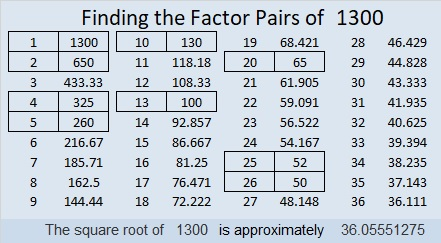# 1300 Prime Factorization for These Hundred Numbers

When I reach a multiple of a hundred, I like to notice how many factors it and the previous group of numbers have. I made this chart so you can see, too.Numbers with 4 factors: 29
Numbers with 8 factors: 17
Numbers with 2 factors: 15
Numbers with 12 factors: 14
Numbers with 16 factors: 8
Numbers with 6 factors: 6

If I made a horserace out of it, FOUR would have taken an early lead, and there would have been no lead changes the entire race. Not very exciting.

Now let me share a few facts about the number 1300:

• 1300 is a composite number.
• Prime factorization: 1300 = 2 × 2 × 5 × 5 × 13, which can be written 1300 = 2² × 5² × 13
• The exponents in the prime factorization are 2, 2 and 1. Adding one to each and multiplying we get (2 + 1)(2 + 1)(1 + 1) = 3 × 3 × 2 = 18. Therefore 1300 has exactly 18 factors.
• Factors of 1300: 1, 2, 4, 5, 10, 13, 20, 25, 26, 50, 52, 65, 100, 130, 260, 325, 650, 1300
• Factor pairs: 1300 = 1 × 1300, 2 × 650, 4 × 325, 5 × 260, 10 × 130, 13 × 100, 20 × 65, 25 × 52 or 26 × 50
• Taking the factor pair with the largest square number factor, we get √1300 = (√100)(√13) = 10√13 ≈ 36.055511300 is the sum of two consecutive prime numbers:
647 + 653 = 1300

OEIS.org informs us that 1⁵ + 2⁵ + 3⁵ + 4⁵ = 1300.

1300 is the sum of two squares THREE different ways:
30² + 20² = 1300
34² + 12² = 1300
36² + 2² =1300

1300 is also the hypotenuse of SEVEN Pythagorean triples:
144-1292-1300 which is 4 times (36-323-325)
320-1260-1300 which is 20 times (16-63-65
364-1248-1300 which is (7-24-25) times 52
500-1200-1300 which is (5-12-13) times 100
660-1120-1300 which is 20 times (33-56-65)
780-1040-1300 which is (3-4-5) times 260
816-1012-1300 which is 4 times (204-253-325)

This site uses Akismet to reduce spam. Learn how your comment data is processed.